ROCKTAPE is the only Kinesiology tape engineered to meet the demands of endurance athletes like runners, swimmers, and cyclists. Unlike other products, ROCKTAPE can be used both to apply compression to promote recovery, or decompression to relieve pain and swelling. Similar to the Kinesiology tape in the Olympics and that Lance Armstong wrote about in “Every Second Counts”, ROCKTAPE is used by professional and amateur athletes everywhere.

Contact Dr. J to order ROCKTAPE

```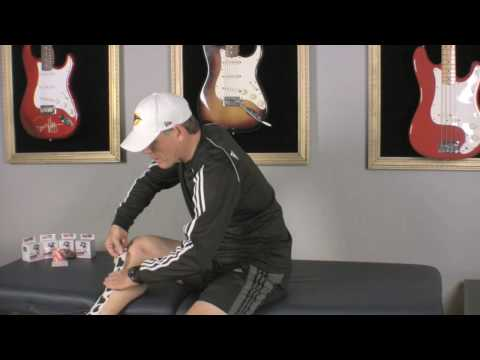/* <![CDATA[ */ jQuery(document).ready(function(\$){ \$(function(){ var width = \$(window).innerWidth(); var setwidth = 640; var ratio = 0.75; var height = 480; var link = 'https://www.youtube.com/watch?v=NHMjhY8Lu98&width=640&height=480'; if(width < setwidth) { height = Math.floor(width * 0.75); //console.log("device width "+width+", set width "+640+", ratio "+0.75+", new height "+ height); var new_url = wpvl_paramReplace('width', link, width); var new_url = wpvl_paramReplace('height', new_url, height); \$("a#62b88a16467b0").attr('href', new_url); //console.log(new_url); } }); }); /* ]]&gt; */ ```Rock Tape for Shin Splints```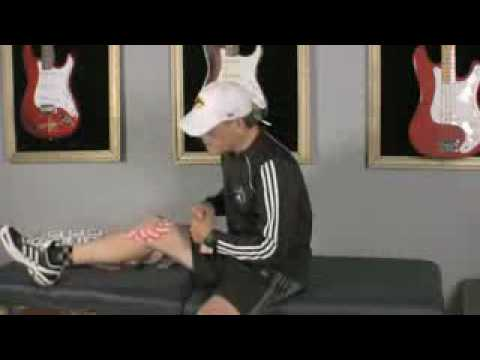/* <![CDATA[ */ jQuery(document).ready(function(\$){ \$(function(){ var width = \$(window).innerWidth(); var setwidth = 640; var ratio = 0.75; var height = 480; var link = 'https://www.youtube.com/watch?v=pvSqqDWs21U&width=640&height=480'; if(width < setwidth) { height = Math.floor(width * 0.75); //console.log("device width "+width+", set width "+640+", ratio "+0.75+", new height "+ height); var new_url = wpvl_paramReplace('width', link, width); var new_url = wpvl_paramReplace('height', new_url, height); \$("a#62b88a16467e9").attr('href', new_url); //console.log(new_url); } }); }); /* ]]&gt; */ ```Rock Tape for Knee

`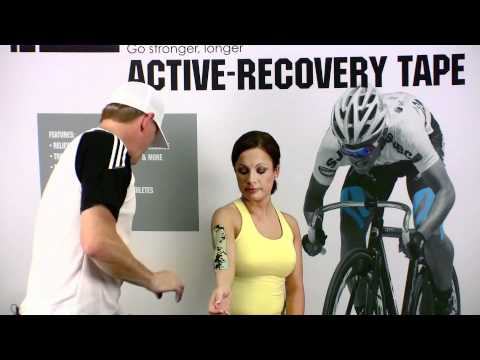`
``` /* <![CDATA[ */ jQuery(document).ready(function(\$){ \$(function(){ var width = \$(window).innerWidth(); var setwidth = 640; var ratio = 0.75; var height = 480; var link = 'https://www.youtube.com/watch?v=M2LKykqqT_8&width=640&height=480'; if(width < setwidth) { height = Math.floor(width * 0.75); //console.log("device width "+width+", set width "+640+", ratio "+0.75+", new height "+ height); var new_url = wpvl_paramReplace('width', link, width); var new_url = wpvl_paramReplace('height', new_url, height); \$("a#62b88a1646810").attr('href', new_url); //console.log(new_url); } }); }); /* ]]&gt; */ ```Rock Tape for Tennis Elbow

`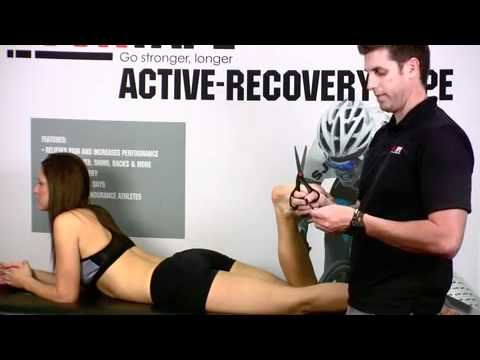`
``` /* <![CDATA[ */ jQuery(document).ready(function(\$){ \$(function(){ var width = \$(window).innerWidth(); var setwidth = 640; var ratio = 0.75; var height = 480; var link = 'https://www.youtube.com/watch?v=0x23gSEqKQ4&width=640&height=480'; if(width < setwidth) { height = Math.floor(width * 0.75); //console.log("device width "+width+", set width "+640+", ratio "+0.75+", new height "+ height); var new_url = wpvl_paramReplace('width', link, width); var new_url = wpvl_paramReplace('height', new_url, height); \$("a#62b88a1646833").attr('href', new_url); //console.log(new_url); } }); }); /* ]]&gt; */ ```Rock Tape for Plantar Fasciitis

`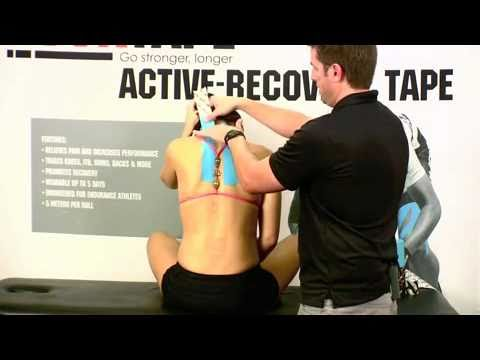`
``` /* <![CDATA[ */ jQuery(document).ready(function(\$){ \$(function(){ var width = \$(window).innerWidth(); var setwidth = 640; var ratio = 0.75; var height = 480; var link = 'https://www.youtube.com/watch?v=0O6QKM13emw&width=640&height=480'; if(width < setwidth) { height = Math.floor(width * 0.75); //console.log("device width "+width+", set width "+640+", ratio "+0.75+", new height "+ height); var new_url = wpvl_paramReplace('width', link, width); var new_url = wpvl_paramReplace('height', new_url, height); \$("a#62b88a1646854").attr('href', new_url); //console.log(new_url); } }); }); /* ]]&gt; */ ```Rock Tape for Neck

```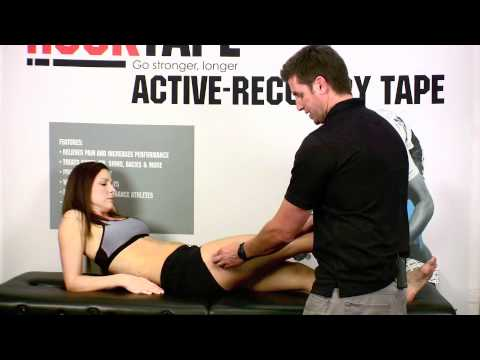/* <![CDATA[ */ jQuery(document).ready(function(\$){ \$(function(){ var width = \$(window).innerWidth(); var setwidth = 640; var ratio = 0.75; var height = 480; var link = 'https://www.youtube.com/watch?v=jL4qcnLQNgA&width=640&height=480'; if(width < setwidth) { height = Math.floor(width * 0.75); //console.log("device width "+width+", set width "+640+", ratio "+0.75+", new height "+ height); var new_url = wpvl_paramReplace('width', link, width); var new_url = wpvl_paramReplace('height', new_url, height); \$("a#62b88a1646873").attr('href', new_url); //console.log(new_url); } }); }); /* ]]&gt; */ ```Rock Tape for IT Band```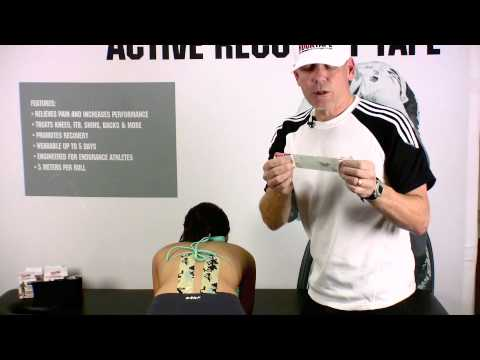/* <![CDATA[ */ jQuery(document).ready(function(\$){ \$(function(){ var width = \$(window).innerWidth(); var setwidth = 640; var ratio = 0.75; var height = 480; var link = 'https://www.youtube.com/watch?v=upnvF5WwQHE&width=640&height=480'; if(width < setwidth) { height = Math.floor(width * 0.75); //console.log("device width "+width+", set width "+640+", ratio "+0.75+", new height "+ height); var new_url = wpvl_paramReplace('width', link, width); var new_url = wpvl_paramReplace('height', new_url, height); \$("a#62b88a1646892").attr('href', new_url); //console.log(new_url); } }); }); /* ]]&gt; */ ```Rock Tape for Lower Back

`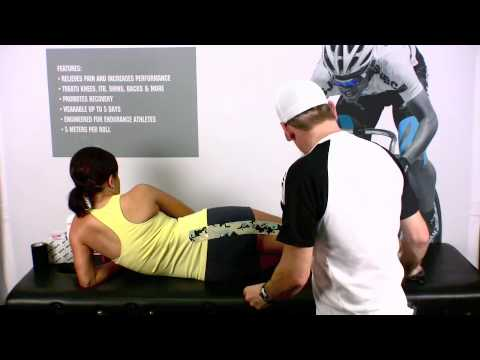`
``` /* <![CDATA[ */ jQuery(document).ready(function(\$){ \$(function(){ var width = \$(window).innerWidth(); var setwidth = 640; var ratio = 0.75; var height = 480; var link = 'https://www.youtube.com/watch?v=00lRDfzpXKc&width=640&height=480'; if(width < setwidth) { height = Math.floor(width * 0.75); //console.log("device width "+width+", set width "+640+", ratio "+0.75+", new height "+ height); var new_url = wpvl_paramReplace('width', link, width); var new_url = wpvl_paramReplace('height', new_url, height); \$("a#62b88a16468b0").attr('href', new_url); //console.log(new_url); } }); }); /* ]]&gt; */ ```Rock Tape for Sciatica

`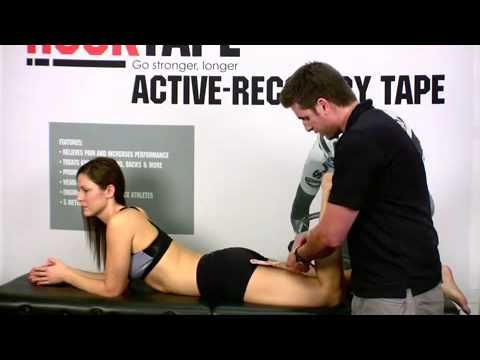`
``` /* <![CDATA[ */ jQuery(document).ready(function(\$){ \$(function(){ var width = \$(window).innerWidth(); var setwidth = 640; var ratio = 0.75; var height = 480; var link = 'https://www.youtube.com/watch?v=3gT_sSlVyxU&width=640&height=480'; if(width < setwidth) { height = Math.floor(width * 0.75); //console.log("device width "+width+", set width "+640+", ratio "+0.75+", new height "+ height); var new_url = wpvl_paramReplace('width', link, width); var new_url = wpvl_paramReplace('height', new_url, height); \$("a#62b88a16468cf").attr('href', new_url); //console.log(new_url); } }); }); /* ]]&gt; */ ```Rock Tape for Calf

`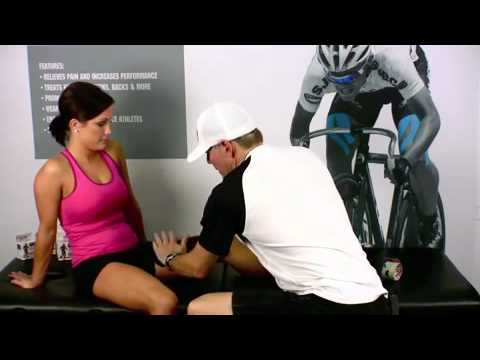`
``` /* <![CDATA[ */ jQuery(document).ready(function(\$){ \$(function(){ var width = \$(window).innerWidth(); var setwidth = 640; var ratio = 0.75; var height = 480; var link = 'https://www.youtube.com/watch?v=fs7hDfUPLfc&width=640&height=480'; if(width < setwidth) { height = Math.floor(width * 0.75); //console.log("device width "+width+", set width "+640+", ratio "+0.75+", new height "+ height); var new_url = wpvl_paramReplace('width', link, width); var new_url = wpvl_paramReplace('height', new_url, height); \$("a#62b88a16468ee").attr('href', new_url); //console.log(new_url); } }); }); /* ]]&gt; */ ```Rock Tape for Groin

```/* <![CDATA[ */ jQuery(document).ready(function(\$){ \$(function(){ var width = \$(window).innerWidth(); var setwidth = 640; var ratio = 0.75; var height = 480; var link = 'https://www.youtube.com/watch?v=amwdo3UicGU&width=640&height=480'; if(width < setwidth) { height = Math.floor(width * 0.75); //console.log("device width "+width+", set width "+640+", ratio "+0.75+", new height "+ height); var new_url = wpvl_paramReplace('width', link, width); var new_url = wpvl_paramReplace('height', new_url, height); \$("a#62b88a1646911").attr('href', new_url); //console.log(new_url); } }); }); /* ]]&gt; */ ```Rock Tape for Rotator Cuff```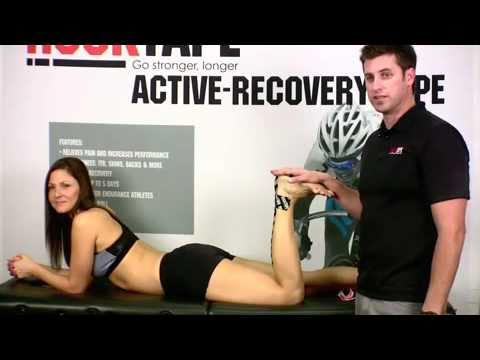/* <![CDATA[ */ jQuery(document).ready(function(\$){ \$(function(){ var width = \$(window).innerWidth(); var setwidth = 640; var ratio = 0.75; var height = 480; var link = 'https://www.youtube.com/watch?v=dLtD8zqZ_m0&width=640&height=480'; if(width < setwidth) { height = Math.floor(width * 0.75); //console.log("device width "+width+", set width "+640+", ratio "+0.75+", new height "+ height); var new_url = wpvl_paramReplace('width', link, width); var new_url = wpvl_paramReplace('height', new_url, height); \$("a#62b88a1646930").attr('href', new_url); //console.log(new_url); } }); }); /* ]]&gt; */ ```Rock Tape for Achilles Tendon

`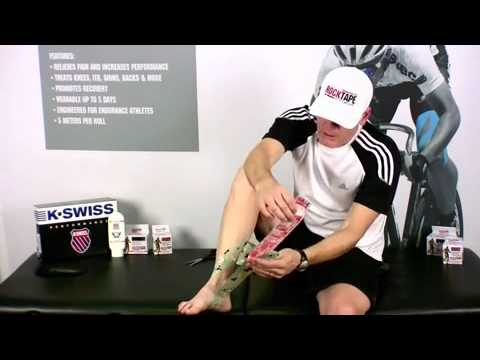`
``` /* <![CDATA[ */ jQuery(document).ready(function(\$){ \$(function(){ var width = \$(window).innerWidth(); var setwidth = 640; var ratio = 0.75; var height = 480; var link = 'https://www.youtube.com/watch?v=Od0NIz78jSA&width=640&height=480'; if(width < setwidth) { height = Math.floor(width * 0.75); //console.log("device width "+width+", set width "+640+", ratio "+0.75+", new height "+ height); var new_url = wpvl_paramReplace('width', link, width); var new_url = wpvl_paramReplace('height', new_url, height); \$("a#62b88a164696f").attr('href', new_url); //console.log(new_url); } }); }); /* ]]&gt; */ ```Rock Tape for Sprained Ankle

`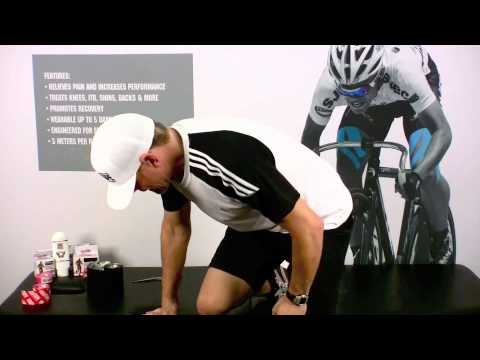`
``` /* <![CDATA[ */ jQuery(document).ready(function(\$){ \$(function(){ var width = \$(window).innerWidth(); var setwidth = 640; var ratio = 0.75; var height = 480; var link = 'https://www.youtube.com/watch?v=vu5DLL8aTEE&width=640&height=480'; if(width < setwidth) { height = Math.floor(width * 0.75); //console.log("device width "+width+", set width "+640+", ratio "+0.75+", new height "+ height); var new_url = wpvl_paramReplace('width', link, width); var new_url = wpvl_paramReplace('height', new_url, height); \$("a#62b88a1646991").attr('href', new_url); //console.log(new_url); } }); }); /* ]]&gt; */ ```Rock Tape for Thigh

`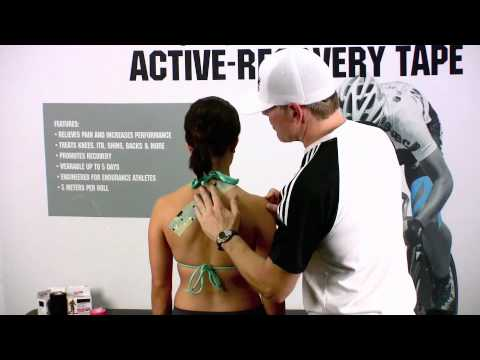`
``` /* <![CDATA[ */ jQuery(document).ready(function(\$){ \$(function(){ var width = \$(window).innerWidth(); var setwidth = 640; var ratio = 0.75; var height = 480; var link = 'https://www.youtube.com/watch?v=-QNCZ_yjnek&width=640&height=480'; if(width < setwidth) { height = Math.floor(width * 0.75); //console.log("device width "+width+", set width "+640+", ratio "+0.75+", new height "+ height); var new_url = wpvl_paramReplace('width', link, width); var new_url = wpvl_paramReplace('height', new_url, height); \$("a#62b88a16469af").attr('href', new_url); //console.log(new_url); } }); }); /* ]]&gt; */ ```Rock Tape for Forward Head Posture